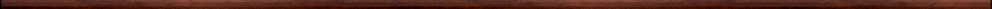Maple worksheets on special inverse functionsNumerical methods topics:

They are all compatible with Classic Worksheet Maple 10.Inverse functions .. I - invfcns1.mws

• Inverse for g(x)=x*exp(x) - the Lambert W function (principal branch)
• Inverse for g(x)=x-arctan(x)
• Inverse for g(x)=x+arctan(x)
• Inverse for g(x)=x*sec(x)

Inverse functions .. II - invfcns2.mws

• Inverse for g(x)=x*cosh(x)
• Inverse for g(x)=sinh(x)-x
• Inverse for g(x)=x+tanh(x)
• Inverse for g(x)=x-tanh(x)

Inverse functions .. III - invfcns3.mws

• Restricted inverses for g(x)=x+sin(x) and h(x)=x-sin(x)
• General inverse for g(x)=x+sin(x)
• General inverse for g(x)=x-sin(x)

Inverse functions .. IV - invfcns4.mws

• Inverse for g(x)=exp(x)-1-x-x^2/2
• Inverse for g(x)=x-arcsinh(x)
• Inverse for g(x)=x+exp(x)

Inverse functions .. V - invfcns5.mws

• The dilogarithm function dilog(x)
• Inverse for g(x)=dilog(x)

Inverse functions .. VI - invfcns6.mws

• Examples involving the special inverse functions
• The "cycloid" function

Inverse function procedures - invfcns.zip

Top of page

Main index